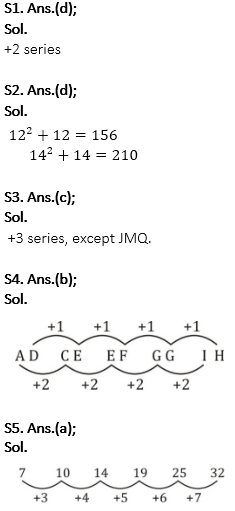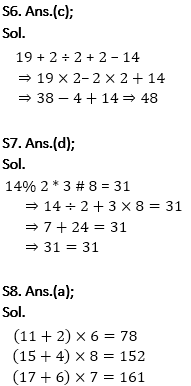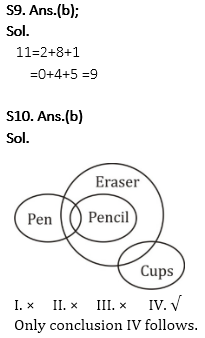Latest SSC jobs   »   Target SSC CGL | 10,000+ Questions...

# Target SSC CGL | 10,000+ Questions | Reasoning Questions For SSC CGL : Day 147

This is the new year with new goals, new experiences and lots of exams to be scheduled soon. SSC CGL has recently released the exam dates so now it is time to gear up your preparations and try hard to get success. ADDA247 never fails to deliver something new and fruitful for you all. This time also we are providing you the best study plan as well as a study material. We are here going to prepare your Reasoning section for the SSC CGL. In this article, we are providing you the details that how we are going to help you to clear the examination this year. We ADDA247 is going to provide you daily tests for all the subjects. The topic-wise quiz will be done from January till May. This will help you to get a deeper knowledge of all the topics and will prepare you thoroughly.

Directions (1-2): In the following question, select the related letters/number from the given alternatives.

Q1. BGMR : DIOT : : SNOV : ?
(a) UPXQ
(b) QPUX
(c) UMPW
(d) UPQX

Q2. 12 : 156 : : 14 : ?
(a) 195
(b) 205
(c) 208
(d) 210

Q3. In the following question, select the odd letters from the given alternatives.
(b) CFI
(c) JMQ
(d) SVY

Q4. A series is given with one term missing. Select the correct alternative from the given ones that will complete the series.
(a) II
(b) IH
(c) HI
(d) IJ

Q5. In the following question, select the missing number from the given series.
7, 10, 14, 19, 25, ?
(a) 32
(b) 36
(c) 38
(d) 40

Q6. If “÷” denotes “subtracted from”, “+” denotes “multiplied by”, “–” denotes “added to” and “×” denotes “divided by”, then
19 + 2 ÷ 2 + 2 – 14 = ?
(a) 52
(b) 46
(c) 48
(d) 88

Q7. If “#” denotes “multiplied by”, “@” denotes “subtracted from”, “*” denotes “added to” and “%” denotes “divided by”, then which of the following equation must be true?
(a) 4 % 2 # 6 * 7 = 18
(b) 3 # 9 @ 4 # 6 = 4
(c) 5 # 6 % 3 @ 4 = 8
(d) 14 % 2 * 3 # 8 = 31

Q8. If 11 # 2 @ 6 = 78 and 15 # 4 @ 8 = 152, then 17 # 6 @ 7 = ?
(a) 161
(b) 143
(c) 221
(d) 157

Q9. If 2 = 0, 5 = 1 and 8 = 4, then 11 = ?
(a) 8
(b) 9
(c) 10
(d) 6

Directions (10): In each of the following question below are given some statements followed by some conclusions. Taking the given statements to be true even if they seem to be at variance from commonly known facts, read all the conclusions and then decide which of the given conclusion logically follows the given statements.

Q10. Statements:
I. Some pens are pencils.
II. All pencils are erasers.
III. Some erasers are cups.
Conclusions:
I. Some pens are cups.
II. Some pencils are cups.
III. Some cups are pencils.
IV. Some erasers are pens.
(a) Only conclusion (II) follows
(b) Only conclusion (IV) follows
(c) Only conclusion (I) follows
(d) No conclusion follows

In the last month i.e. in May daily we will provide you with a test of the previous years’ question papers, this will increase your confidence of solving the real exam and will make you familiar with the real-time exam.

Solutions#### Congratulations!

General Awareness & Science Capsule PDF